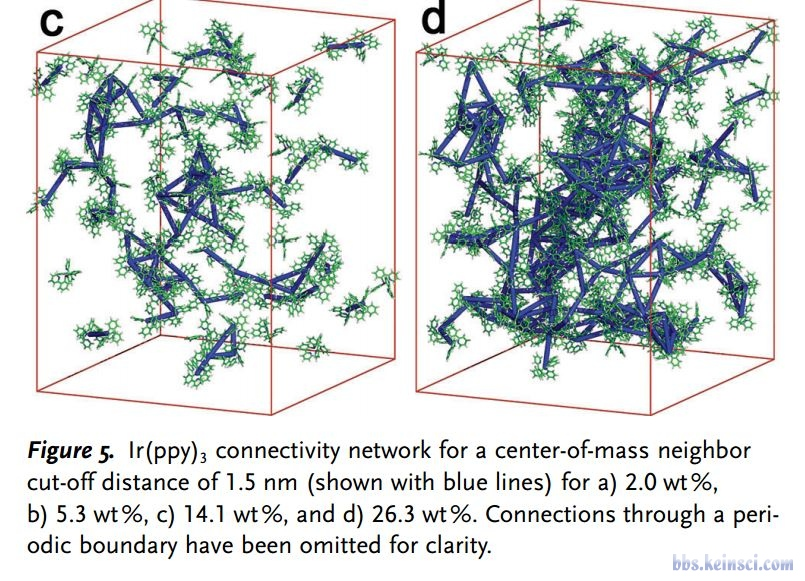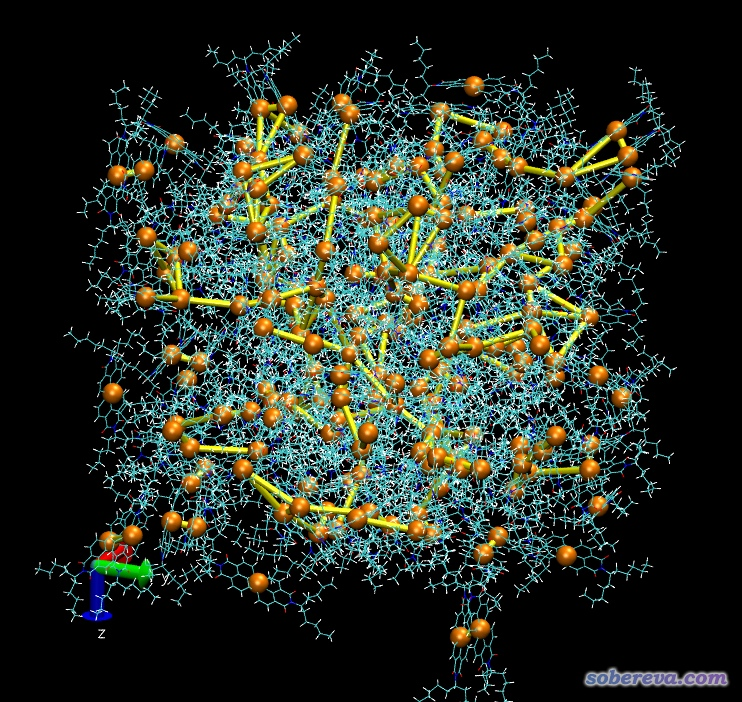# 在VMD中将距离较近的分子质心连线的脚本draw delete all
draw color orange
set nres [llength [lsort -unique [[atomselect top all] get residue]]]
for { set ires 1 } { \$ires <= \$nres } { incr ires } {
set sel [atomselect top "resid \$ires"]
set COM [measure center \$sel weight mass]
set x(\$ires) [lindex \$COM 0]
set y(\$ires) [lindex \$COM 1]
set z(\$ires) [lindex \$COM 2]
draw sphere "\$x(\$ires) \$y(\$ires) \$z(\$ires)" radius 1.5
\$sel delete
}

draw color yellow
set crit 10
for { set ires 1 } { \$ires <= \$nres } { incr ires } {
for { set jres [expr \$ires+1] } { \$jres <= \$nres } { incr jres } {
set dx2 [expr (\$x(\$ires)-\$x(\$jres))**2]
set dy2 [expr (\$y(\$ires)-\$y(\$jres))**2]
set dz2 [expr (\$z(\$ires)-\$z(\$jres))**2]
set dist [expr sqrt(\$dx2+\$dy2+\$dz2)]
if { \$dist<\$crit } {
draw cylinder "\$x(\$ires) \$y(\$ires) \$z(\$ires)" "\$x(\$jres) \$y(\$jres) \$z(\$jres)" radius 0.5
}
}
}

## 用法：